# Probability with Spinners

Probability with Spinners• The sample space is the list of all possible outcomes that the spinner can land on.
• We will write the probability of spinning a 1 as a fraction.
• This probability is equal to the amount of ‘1’s divided by the total amount of numbers on the spinner.
• There are 8 numbers in total on the spinner.
• There are 3 ones on the spinner.
• The probability of spinning a ‘1’ is   3 / 8   .
• The spinner will land on a ‘1’ three times out of every eight.

The probability of the spinner landing on a number is equal to the fraction of the spinner that this number occupies.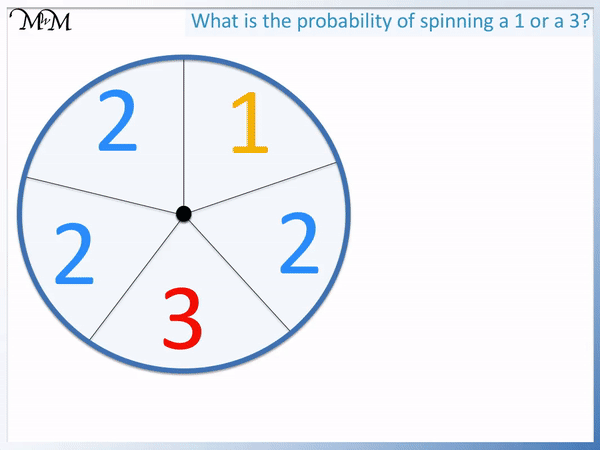• We will write the probability of spinning a ‘1’ or a ‘3’ as a fraction.
• This spinner is split into 5 equal sections.
• One section contains a ‘1’ and another section contains a ‘3’.
• 2 out of the 5 sections on the spinner contain a ‘1’ or a ‘3’.
• We can write the probability as   2 / 5   .
• Because 2 sections is less than half of the spinner, the spinner will land on a ‘1’ or a ‘3’ less than half of the time.
• The probability of spinning a ‘1’ or a ‘3’ is therefore unlikely# Probability with Spinners

Below is a spinner. The arrow is spun and the number that it lands on is the score that we receive. This number is called the outcome.The list of all possible outcomes is called the sample space.

We make a list of all of the numbers that the spinner can land on and we do not need to write each outcome more than once.The sample space for this spinner is:

1, 2, 3 and 5

Probability measures how likely it is that something will happen.

We can write probability in words, as a decimal, a percentage or a fraction. We tend to write whichever is easiest

In this lesson we will be writing the probability as a fraction because spinners are divided into equal sections.

In the example below we are asked, “What is the probability of rolling a 1?”.

We can write probability as:   the number of desired outcomes / the number of total outcomes   .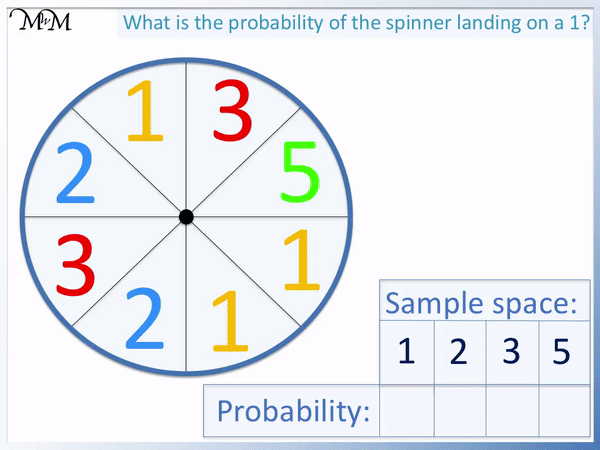There are 8 sections on the spinner in total. So the number of total outcomes is 8.

We write our probability as a fraction out of 8.

Now we count how many of these 8 sections contain a ‘1’.

There are 3 ones on the spinner.

3 out of 8 parts contain a ‘1’.

The probability of spinning a 1 is   3 / 8   .

This means that we can expect the spinner to spin a ‘1’ three times if it is spun eight times.

We will now look at the probability of spinning a ‘2’.There are 2 sections on the spinner that contain a ‘2’.

The probability of spinning a 2 is   2 / 8   .

The probability of spinning a 3 is also   2 / 8   .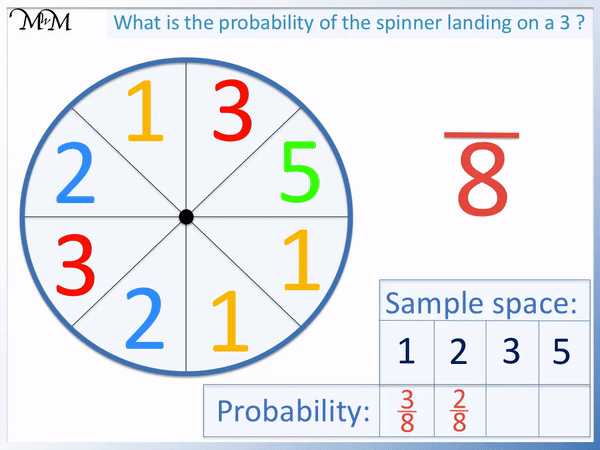Spinning a 2 and spinning a 3 are equally likely on this spinner because there is the same probability of each outcome occurring.

The probability of spinning a 5 is   1 / 8   .We have filled our sample space with the probabilities of spinning each outcome.

We can check that our fractions all add up to   8 / 8   or one whole.

We can see that   2 / 8   can be simplified to   1 / 4   .

This means that we can expect a 2 a quarter of the times that the spinner is spun.

We can also expect a 3 a quarter of the times that the spinner is spun.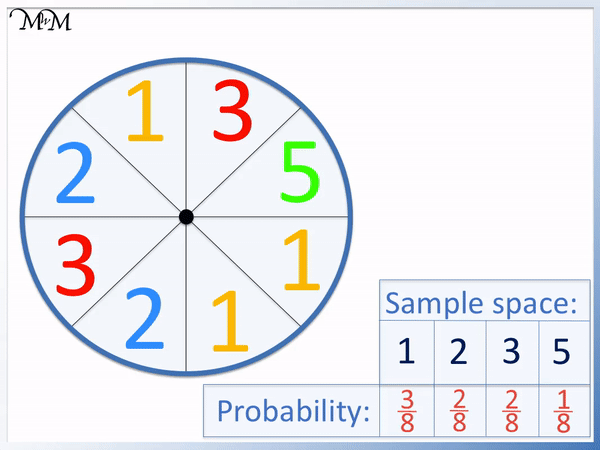We can show the probabilities in our sample space on a probability scale.

The probability scale is a line drawn between zero and one.

A probability of zero means that there is zero chance of the outcome occurring.

Something with a probability of zero is impossible.

A probability of 1 whole means that the outcome is certain.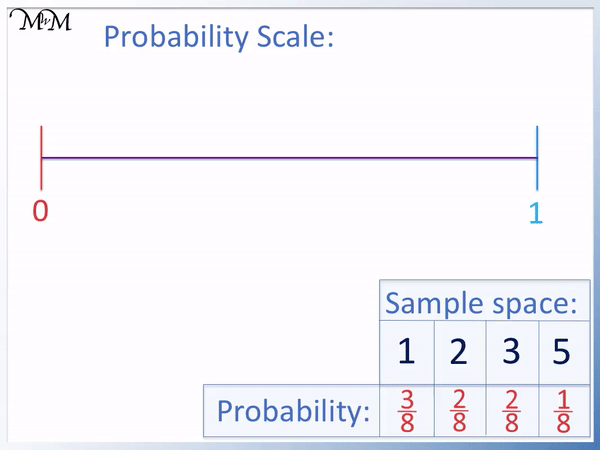A probability of one half is in the middle of zero and one.

A probability of one half means that the outcome occurs half of the time.

We can use this to describe our outcomes.

Anything that occurs less than half of the time we describe as unlikely.

Anthing that occurs more than half of the time we describe as likely.

The outcomes of the spinner are all fractions out of 8.

We can label their positions on the probability scale by dividing the scale into eight equal parts.We can see that spinning a 1 is the most likely outcome because it has the biggest probability of occurring.

When teaching probability, a common misconception is that if something is the most likely then we will expect it to occur most of the time.

However we can see that all of the outcomes are unlikely to occur because they are all less than   1 / 2   .

Below is a new example with a spinner divided into 5 equally sized sections.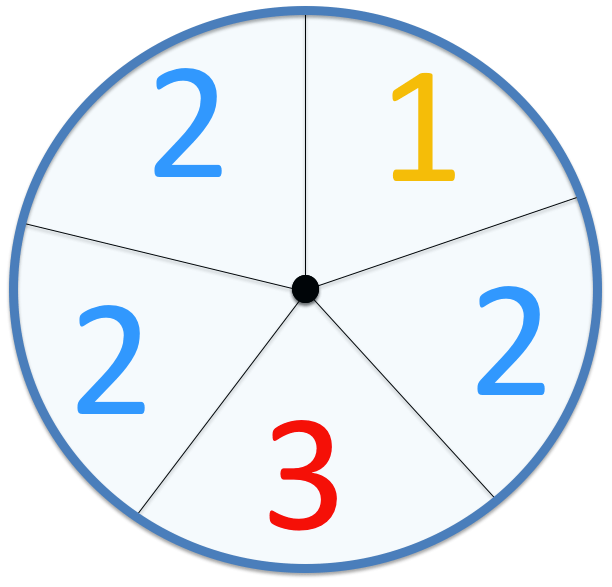The probability of spinning a one can be written as a fraction.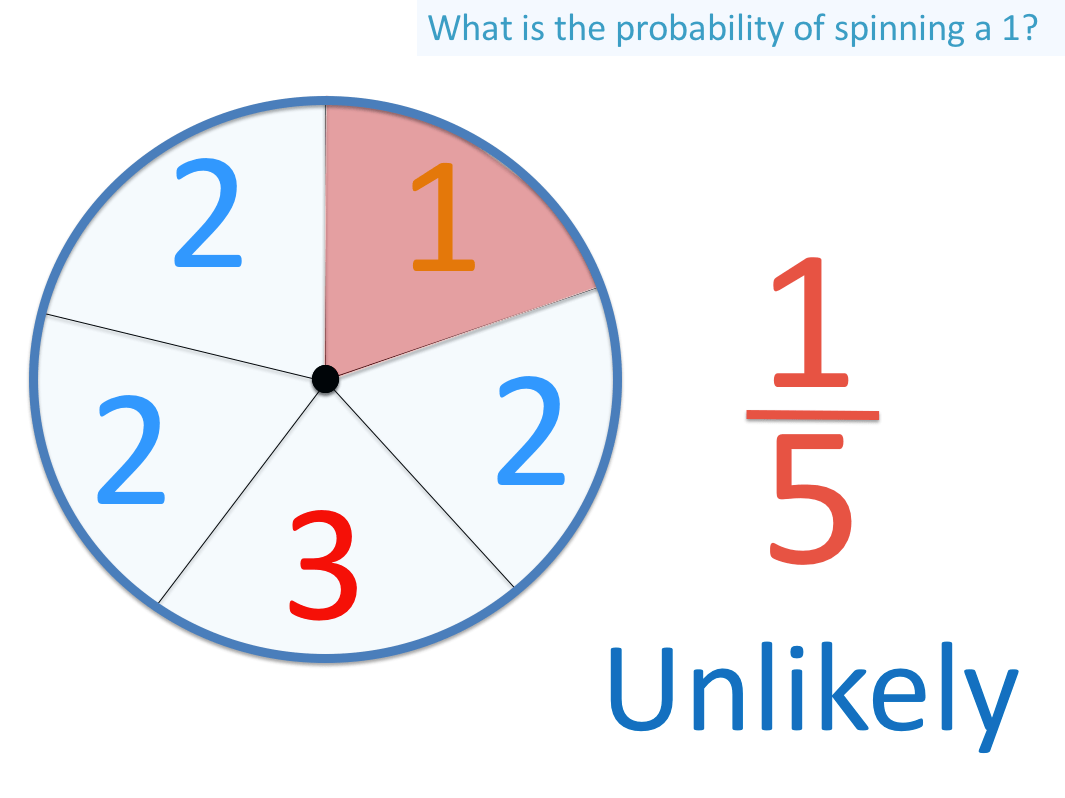One out of five sections contain a ‘1’ and so, the probability of spinning a one is   1 / 5   .

Because 1 is less than half of 5, this outcome can be described as unlikely.

The probability of spinning a two is   3 / 5   .Because this fraction is greater than one half, this outcome can be described as likely.

The following example asks, “What is the probability of spinning a 1 or a 3?”.

We look at how many outcomes are either a ‘1’ or a ‘3’.

There are two sections on the spinner that are a ‘1’ or a ‘3’.The probability of spinning a 1 or a 3 is   2 / 5   .

Two is less than half of five and so this outcome is unlikely.In the example below we are asked for the probability of spinning a number greater than 1.

The numbers 2 and 3 are both greater than 1.

We cannot include 1 as we are asked for numbers that are strictly greater than 1.There are four sections on the spinner that contain numbers greater than 1.

The probability of spinning a number greater than 1 is   4 / 5   .

Since this fraction is much larger than one half, we can describe the outcome as very likely.Now try our lesson on Probability with Dice where we learn about probability with dice.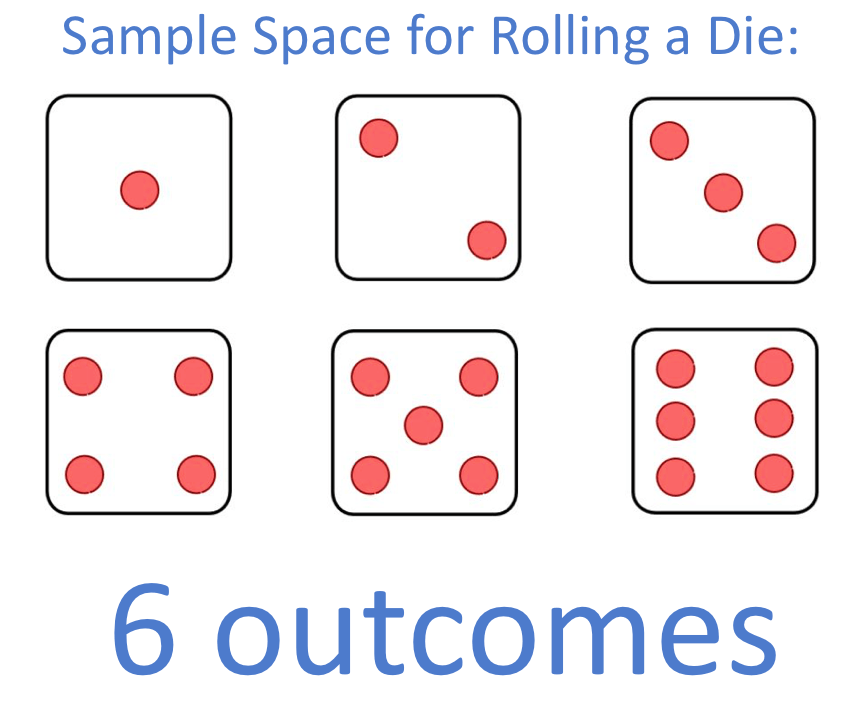error: Content is protected !!